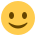#### Howdy, Stranger!

It looks like you're new here. If you want to get involved, click one of these buttons!

Supported by

# BayesFactor: Scale of Cauchy prior in t tests and ANOVA

Hi, I'd like to apologize in advance, if I missed a note regarding the following behavior in the documentation or if this question has been asked before.

After reading about the ANOVA model implemented in the BayesFactor package (Rouder, Morey, Speckman, & Province, 2012) a while ago I wondered whether the scale for the Cauchy prior is affected by centering the design matrix (I should say that I don't fully understand the process of projecting the matrix into the lower dimensional space and its implications). As discussed in the paper (p. 363), in case of two groups in which groups are coded as 0.5 and -0.5, the projected matrix yields a coding of sqrt(2)/2 and -sqrt(2)/2. As a result, the effect is not coded as a distance of 1 but rather as a distance of sqrt(2). Unless I misunderstand, this implies that the Cauchy prior for fixed effects in the ANOVA needs to be scaled accordingly; otherwise r = 1 would imply a different prior in the case of the t test (where no design matrix is centered) and the ANOVA.

I tried this in the BayesFactor package and it appears that this is indeed the case:

``````library("BayesFactor")
library("dplyr")

medium_scale <- sqrt(2) / 2
wide_scale <- 1
ultrawide_scale <- sqrt(2)

ttest_medium <- ttestBF(formula = extra ~ group, data = sleep, rscale = medium_scale)
ttest_wide <- ttestBF(formula = extra ~ group, data = sleep, rscale = wide_scale)
ttest_ultrawide <- ttestBF(formula = extra ~ group, data = sleep, rscale = ultrawide_scale)

# Different results despite same r
ttest_medium %>%
as.vector ==
anovaBF(formula = extra ~ group, data = sleep, rscaleFixed = medium_scale) %>%
as.vector

ttest_wide %>%
as.vector ==
anovaBF(formula = extra ~ group, data = sleep, rscaleFixed = wide_scale) %>%
as.vector

ttest_ultrawide %>%
as.vector ==
anovaBF(formula = extra ~ group, data = sleep, rscaleFixed = ultrawide_scale) %>%
as.vector

# Same results when r is scaled to fit projected design matrix
distance <- BayesFactor:::fixedFromRandomProjection(nlevRandom = 2) %>%
as.vector %>%
diff

ttest_medium %>%
as.vector ==
anovaBF(formula = extra ~ group, data = sleep, rscaleFixed = medium_scale / distance) %>%
as.vector

ttest_wide %>%
as.vector ==
anovaBF(formula = extra ~ group, data = sleep, rscaleFixed = wide_scale / distance) %>%
as.vector

ttest_ultrawide %>%
as.vector ==
anovaBF(formula = extra ~ group, data = sleep, rscaleFixed = ultrawide_scale / distance) %>%
as.vector
``````

I find this quite confusing, as I was under the impression that the scale of the (multivariate) Cauchy in the ANOVA case was similarly placed on the scale of standardized mean differences (d) for each effect. However, from toying around with this example I get the impression that the prior is placed on scaled d units. Is this correct? Does the centering of the matrix cause similar changes to the scale of the prior for each mean difference within a factor? How do I correct for them, if I want to use a consistent prior across analyses?

Frederik

• For the record, the answer by Richard is here:

Richard: "if X is the t-test prior scale, then X/sqrt(2) is the ANOVA scale, and X/2 is the corresponding regression scale."

You: "Thanks for clarifying. So this is not directly related to the rescaled distances between factor levels in the centered design matrix? I think I need to play around with this some more."

Richard: "Yes, it is. The ANOVA model uses orthonormal contrasts, while the t test didn't (e.g., -.5, .5 does not have length 1, but (-sqrt(2)/2, sqrt(2)/2) does)"

You: "Thanks again, I think I understand now. If I want to use a (multivariate) Cauchy(r = x)-prior I need to specify rscaleFixed = x/sqrt(2) or rscaleCont = x/2. I think, a note to this effect in the documentation would be helpful. This seems unobvious to me. (cc @EJWagenmakers)"
:-)

• edited January 2018

Thanks, you beat me to it.